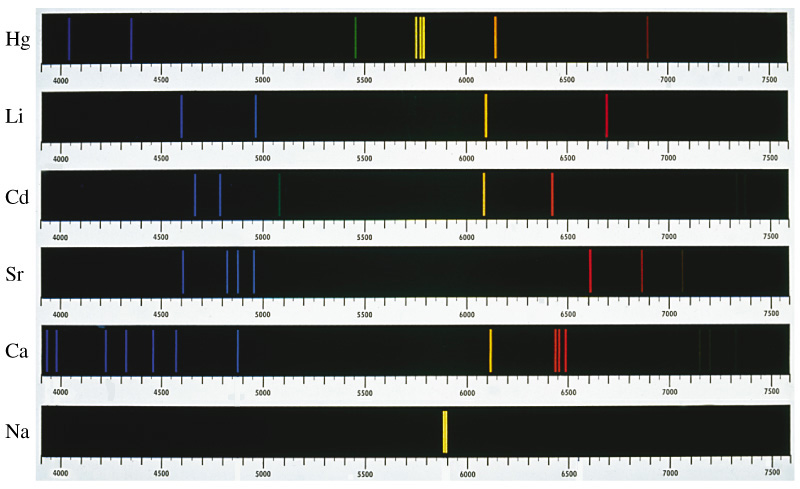# Question #77683

Feb 16, 2014

The Bohr Theory explains flame colours qualitatively but not quantitatively.

#### Explanation:

The Bohr model says that electrons exist only at discrete energy levels.

When an electron drops from a higher to a lower energy level, it emits a quantum of energy.

f the emitted quanta are in the energy range that is visible to our eyes, we see them as light of a particular colour.

The Bohr model explains the line spectrum of hydrogen. The reason is that it involves only the interactions between one electron and the nucleus. We can calculate the exact wavelengths of the emissions.In H atoms, every level with the same quantum number n has the same energy.

In atoms with more than one electron, this is not the case. The electrons repel each, and this changes their energy levels.

Electrons with the same quantum number n no longer have the same energies. Even a helium atom has a much more complicated spectrum than hydrogen.The energy level diagrams are more complicated for larger atoms. So we can’t use Bohr Theory to calculate the exact wavelengths (colours) of the emissions from flame tests.

Even so, the electrons in the atoms know their own energy levels. Each element gives a characteristic flame emission spectrum as its excited electrons drop to lower levels.Here is a video summarizing a flame test experiment.

Video from: Noel Pauller